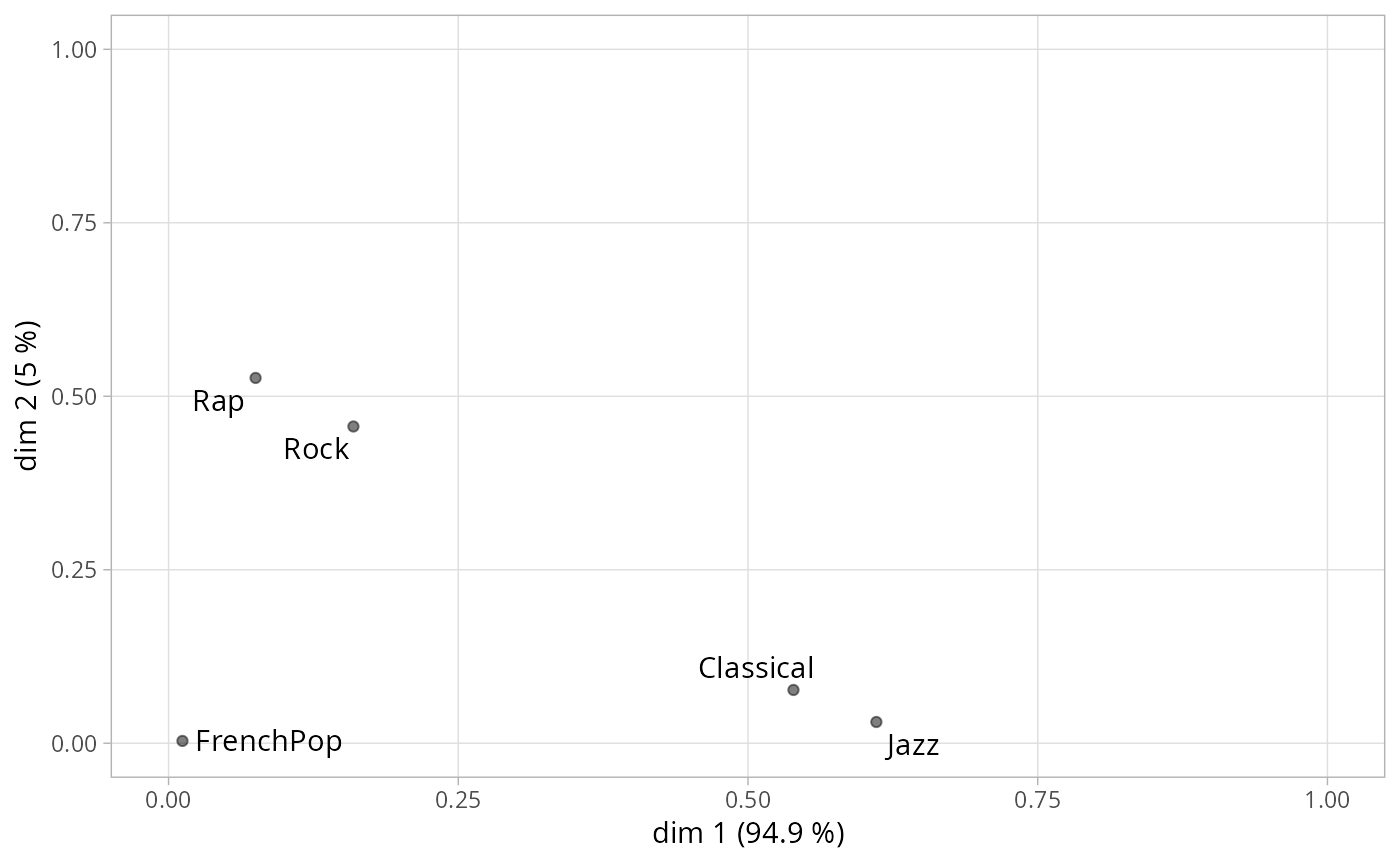Plots the eta-squared (squared correlation ratios) of the active variables of a MCA.

ggeta2_variables(resmca, axes = c(1,2))

## Arguments

resmca

object of class MCA, speMCA, csMCA, stMCA or multiMCA.

axes

numeric vector of length 2, specifying the components (axes) to plot. Default is c(1,2).

## Details

This plot was proposed by Escofier and Pagès (2008) under the name "carré des liaisons", i.e. square of relationships, using correlation ratios to measure these relationships. Eta-squared (i.e. correlation ratio) is a measure of global association between a continuous variable and a categorical variable : it measures the share of variance of the continuous variables "explained" by the categorical variable. Here, it is used to plot the association between the active variables and the axes of the MCA cloud.

## Value

a ggplot2 object

## Author

Nicolas Robette

ggcloud_variables, ggadd_attractions
data(Music)